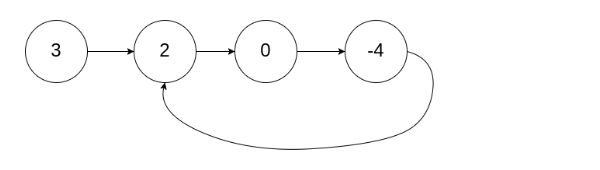New update is available. Click here to update.

# Detect And Remove Cycle

Contributed by
Sakshi Bansal
Last Updated: 23 Feb, 2023
Easy0/40
Avg time to solve 10 mins
Success Rate 90 %Share33 upvotes

## Problem Statement

Suggest Edit

#### A cycle occurs when a node's ‘next’ points back to a previous node in the list.

Detailed explanation ( Input/output format, Notes, Images )##### Constraints :
``````1 <= T <= 10
1 <= N <= 5 * 10^4
-1 <= pos < N
-10^9 <= data <= 10^9 and data != -1

Where 'N' is the size of the singly linked list, and "data" is the Integer data of the singly linked list.
``````
##### Sample Input 1 :
``````2
3 2 0 -4 -1
1
1 2 -1
-1
``````
##### Sample Output 1 :
``````True
3 2 0 -4 -1
False
1 2 -1
``````
##### Explanation for Sample Input 1 :
``````In the 1st test case, there is a cycle, from index 1 -> 2 -> 3 -> 1. The cycle's length is 3.
````````````In the 2nd test case, there is no cycle.
``````
##### Sample Input 2 :
``````2
1 1 1 -1
0
3 -3 -1
-1
``````
##### Sample Output 2 :
``````True
1 1 1 -1
False
3 -3 -1
``````AutoConsole